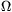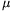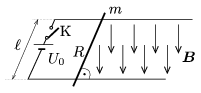Mathematical and Physical Journal
for High Schools
Issued by the MATFUND Foundation
 Already signed up? New to KöMaL?

#Problem P. 4271. (September 2010)

P. 4271. There is a pair of parallel, horizontal, conducting rails in uniform vertically downward magnetic field of induction B=0.5 T. The two rails are at a distance of=10 cm and they are connected to a rechargeable battery of potential difference U0=12 V. A metal rod of mass m=50 g, and of resistance R=2, is placed onto the rails, perpendicularly to them. The coefficient of friction between the rod and the rails is=0.3.

a) What is the initial acceleration of the rod when switch K is closed?

b) What would the final speed of the rod be if the rails are very long?

c) Estimate, (try to give a precise estimation) the covered distance, and the time during which the speed of the rod increases fromto.(5 pont)

Deadline expired on October 11, 2010.

Sorry, the solution is available only in Hungarian. Google translation

Megoldás. $\displaystyle a)$ A kezdeti gyorsulás $\displaystyle 3~m/s^2$.

$\displaystyle b)$ A rúd végsebessége 120 m/s lehet.

$\displaystyle c)$ 34 ms alatt 14 cm-es úton gyorsul fel a rúd 4,0 m/s-ról 4,1 m/s sebességre.

### Statistics:

 76 students sent a solution. 5 points: Ayhan Dániel, Bánhidi Dávid, Barta Szilveszter Marcell, Batki Bálint, Béres Bertold, Czigány Máté Gábor, Dávid Péter, Filep Gábor, Gál Szabolcs, Gargauly Gergő János, Herczeg Ferenc, Jéhn Zoltán, Jenei Márk, Kóbor Attila, Kovács 444 Áron, Köpenczei Gergő, Laczkó Zoltán Balázs, Lőrincz Dóra, Maknics András, Nagy 111 Miklós, Nagy Dániel Bálint, Nagy Lajos, Németh Gergely, Pázmán Koppány, Prok Tamás, Schwarcz Gergő, Seress Dániel, Szabó 928 Attila, Szélig Áron, Szemes Gábor Bence, Szikszai Lőrinc, Szöllősi Márton, Tamási Mátyás, Varga 515 Balázs, Vargha Sára, Várnai Péter, Vuchetich Bálint. 4 points: 19 students. 3 points: 8 students. 2 points: 3 students. 1 point: 7 students. 0 point: 2 students.

Problems in Physics of KöMaL, September 2010